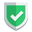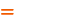我们相信：世界是美好的，你是我也是。平行空间的世界里面，不同版本的生活也在继续...

## 问题重现

``````import numpy as np

s = np.array([list("苏南大叔"), ["su", "nan", "da", "shu"]])
s_ = s
s1 = s
s2 = s
s = "苏"
s = "da"
print(s, type(s))      # 本应该被修改的原版， <class 'numpy.ndarray'>
print(s_, type(s_))    # 不应该被修改的盗版， <class 'numpy.ndarray'>
print(s1, type(s1))    # 不应该被修改的目标切片，['苏' '苏' '苏' '苏']，<class 'numpy.ndarray'>
print(s2, type(s2))    # 不应该被修改的目标切片【实际没被修改】，大 <class 'numpy.str_'>``````

``````[['苏' '南' 'da' '叔']
['苏' '苏' '苏' '苏']] <class 'numpy.ndarray'>
[['苏' '南' 'da' '叔']
['苏' '苏' '苏' '苏']] <class 'numpy.ndarray'>
['苏' '苏' '苏' '苏'] <class 'numpy.ndarray'>

• 作为备份的`s_`被修改了，【不符合预期】，`numpy.ndarray`类型。
• 作为切片的`s1`被修改了，【不符合预期】，`numpy.ndarray`类型。
• 作为切片的`s2`没有被修改，【符合预期】，`numpy.str_`类型。

`s1``s2`同时作为切片，一个被修改另外一个确没有被修改，最大的主因就是数据类型。只要是数据类型是`ndarray`，使用等号建立的新变量，就是引用，就存在着问题。

## 解决方案

``````import numpy as np

s = np.array([list("苏南大叔"), ["su", "nan", "da", "shu"]])
s_ = s.copy()
s1 = s.copy()
s2 = s.copy()
s = "苏"
s = "da"
print(s, type(s))      # 本应该被修改的原版， <class 'numpy.ndarray'>
print(s_, type(s_))    # 不应该被修改的盗版【实际没被修改】， <class 'numpy.ndarray'>
print(s1, type(s1))    # 不应该被修改的切片【实际没被修改】，['su' 'nan' 'da' 'shu']，<class 'numpy.ndarray'>
print(s2, type(s2))    # 不应该被修改的切片【实际没被修改】，大 <class 'numpy.str_'>``````

``````[['苏' '南' 'da' '叔']
['苏' '苏' '苏' '苏']] <class 'numpy.ndarray'>
[['苏' '南' '大' '叔']
['su' 'nan' 'da' 'shu']] <class 'numpy.ndarray'>
['su' 'nan' 'da' 'shu'] <class 'numpy.ndarray'>

## 字符串`.copy()`

``````import numpy as np
s = np.str_("苏南大叔")
s_ = s.copy()
s2 = "苏南大叔"
s3 = s2.copy()``````

``````Traceback (most recent call last):
File "C:\Users\sunan\Desktop\demo\py\t.py", line 4, in <module>
s3 = s2.copy()
AttributeError: 'str' object has no attribute 'copy'``````

## 结束语如果本文对您有帮助，或者节约了您的时间，欢迎打赏瓶饮料，建立下友谊关系。本博客不欢迎：各种镜像采集行为。请尊重原创文章内容，转载请保留作者链接。【福利】 腾讯云最新爆款活动！1核2G云服务器首年50元！【源码】本文代码片段及相关软件，请点此获取更多信息【绝密】秘籍文章入口，仅传授于有缘之人python